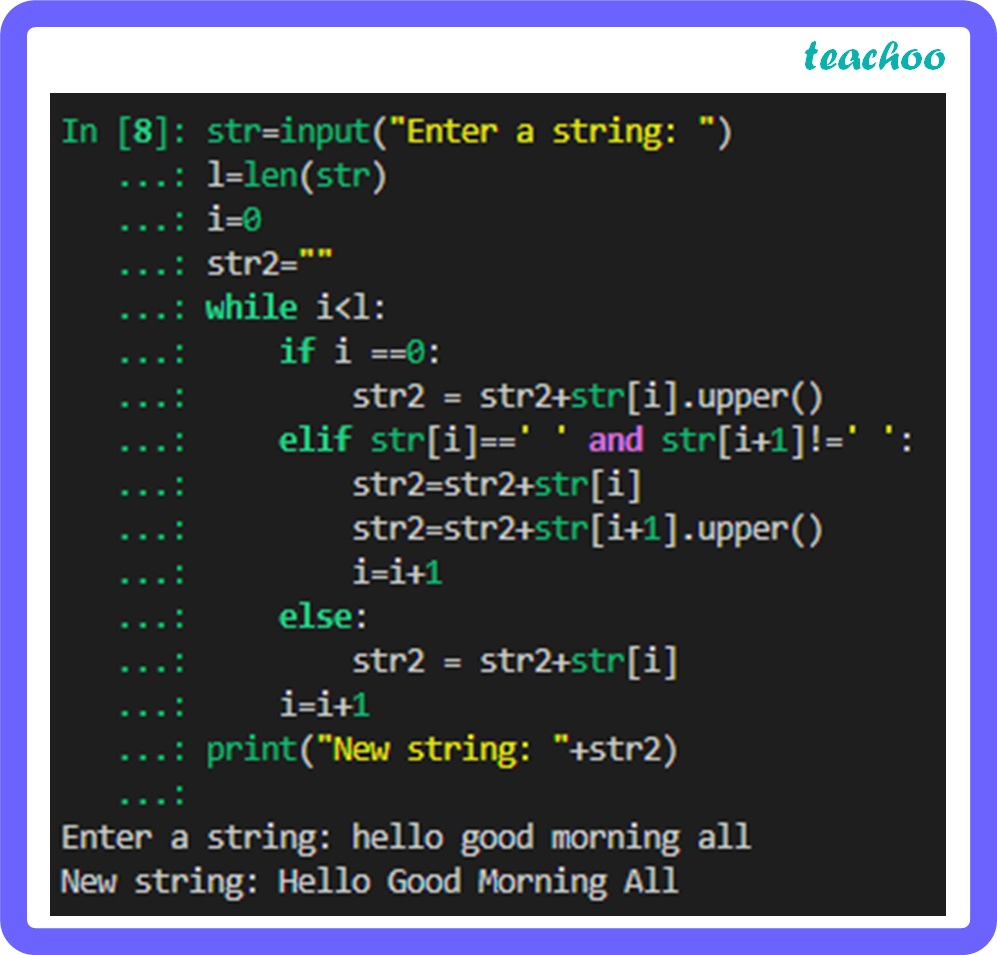Long Answer Type Questions (5 Marks each)

Computer Science - Class 11
Chapter 8 Class 11 - Strings

## Write a program that takes a string with multiple words and capitalizes the first letter of each word and forms a new string out of it.Code:

``` str=input( "Enter a string: " ) ```

``` l=len(str) ```

``` i= 0 ```

``` str2= "" ```

``` while i<l: ```

```     if i == 0 : ```

```         str2 = str2+str[i].upper() ```

```     elif str[i]== ' ' and str[i+ 1 ]!= ' ' : ```

```         str2=str2+str[i] ```

```         str2=str2+str[i+ 1 ].upper() ```

```         i=i+ 1 ```

```     else: ```

```         str2 = str2+str[i] ```

```     i=i+ 1 ```

``` print( "New string: " +str2)  ```

Explanation:

The first letter of the string and every letter after a blank space is converted to uppercase using the upper( ) method.

Learn in your speed, with individual attention - Teachoo Maths 1-on-1 Class